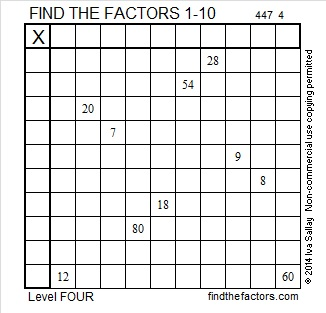# 306 and Level 4

• 306 is a composite number.
• Prime factorization: 306 = 2 x 3 x 3 x 17, which can be written 306 = 2 x 3² x 17
• The exponents in the prime factorization are 1, 2, and 1. Adding one to each and multiplying we get (1 + 1)(2 + 1)(1 + 1) = 2 x 3 x 2 = 12. Therefore 306 has exactly 12 factors.
• Factors of 306: 1, 2, 3, 6, 9, 17, 18, 34, 51, 102, 153, 306
• Factor pairs: 306 = 1 x 306, 2 x 153, 3 x 102, 6 x 51, 9 x 34, or 17 x 18
• Taking the factor pair with the largest square number factor, we get √306 = (√9)(√34) = 3√34 ≈ 17.493Happy Thanksgiving!Print the puzzles or type the factors on this excel file: 10 Factors 2014-11-24

Here’s a little more about the number 306:

306 = 17 × 18, which means it is the sum of the first 17 even numbers.

• Thus, 2 + 4 + 6 + 8 + . . .  + 30 + 32 + 34 = 306

It also means that we are halfway between 17² and 18², or halfway between 289 and 324. The average of those two numbers is 306.5.

AND it means that 17² + 18² – 1 = 2(306) = 2(17 × 18)## One thought on “306 and Level 4”

1.Steve Morris

Happy Thanksgiving to you too!

This site uses Akismet to reduce spam. Learn how your comment data is processed.